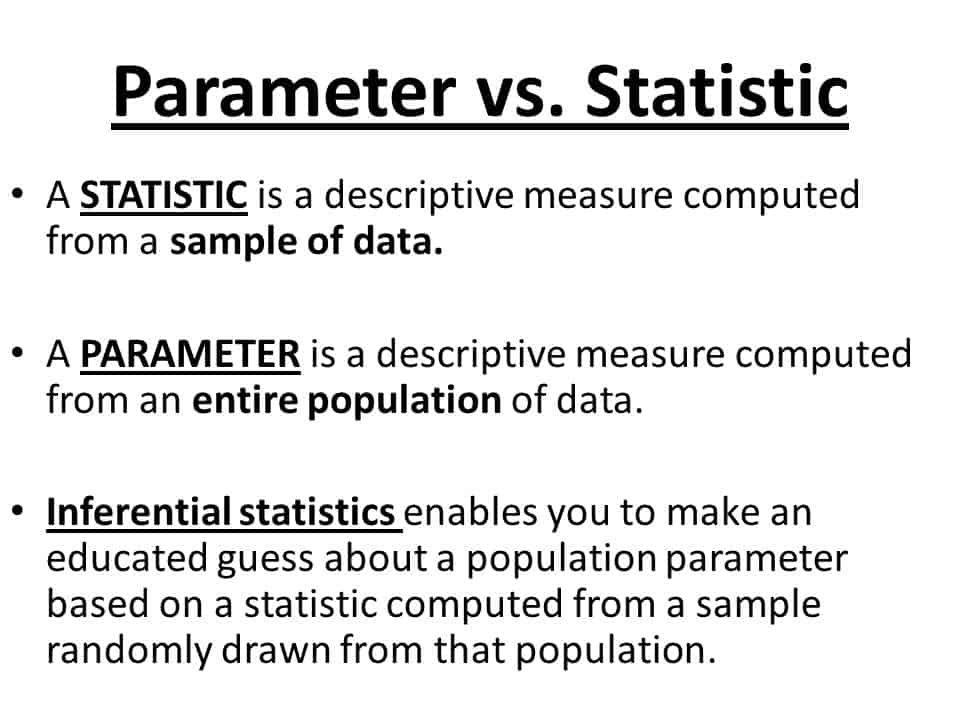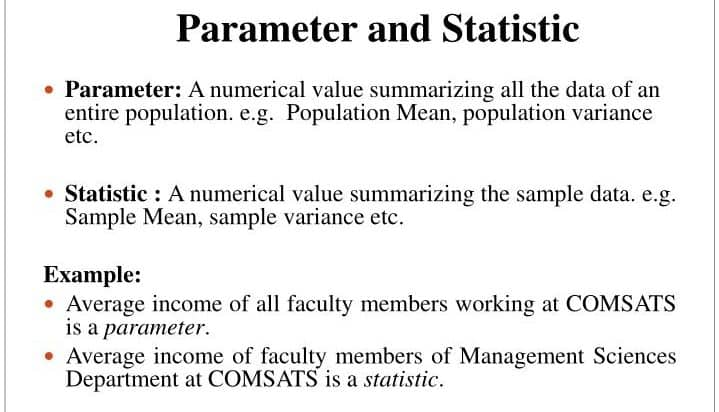Latest Articles
Home / Business Statistics / What is the difference between Parameter and Statistic?

Page Contents

# What is the difference between Parameter and Statistic?

What is the difference between Parameter and Statistic?

A statistic and a parameter are quite similar. They are both describing any of particular groups, like “50% of homeowners prefer Y brand home developers.” The difference between a statistic and a parameter is that statistics describe a sample. A parameter describes an entire population.

Difference between Parameter and Statistic are showing below.

 S.N. Parameter Statistic 01 Any function of the population values is called the parameter. Any function of the sample observation is called statistics. 02 The parameter is an unknown constant. The statistic does not contain unknown constant. 03 Parameters are not used to estimate population characteristics. Statistics are used to estimate population characteristics  ( such as parameters). 04 Parameters are free from sampling and other errors. Statistics are subject to sampling and non-sampling error. 05 There is no distribution of parameters. Statistics have distribution,  which is called sampling distribution. 06 The population mean μ, Variance σ2    etc are called parameter. The sample mean, variance σ2, etc. are called statistics. 07 In the case of parameters, the Greek letter “mu” represents the population mean. In the case of statistics, the “x-bar” symbol represents the sample mean, and most other notations differ too.

Here, Summarized Differences are mentioned.Here, Summarized Differences are mentioned in showing an example.Symbolic expressions related to Parameter and Statistic.## Estimator.

When Statistics is used to estimate a parameter is called an estimator.

## Estimate.

Any particular value of an estimator is called an estimate.Photo Credit

Did our post-effective to help you see the exact differences between parameter and statistic? Please comment below what you learned from overall resources.